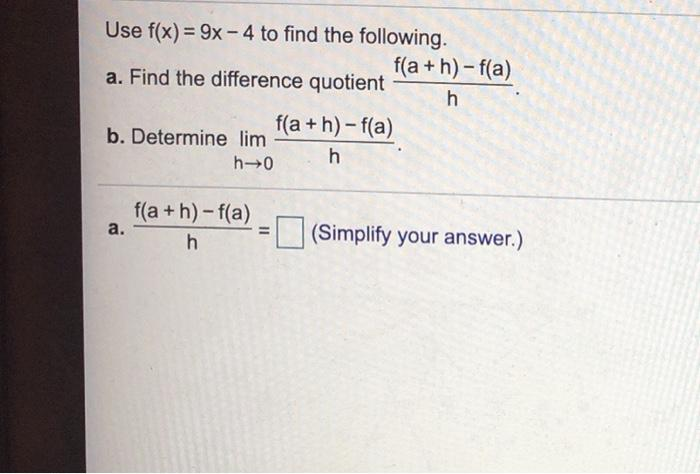### Create an Account

Already have account?

### Forgot Your Password ?

Home / Questions / Use f(x) = 9x - 4 to find the following. f(a+h)-f(a) a. Find the difference quotient h f(a...

# Use f(x) = 9x - 4 to find the following. f(a+h)-f(a) a. Find the difference quotient h f(a+h)-f(a) b. Determine lim h→0 h f(a+h)-f(a) a. h II (Simplify your answer.)

Use f(x) = 9x - 4 to find the following. f(a+h)-f(a) a. Find the difference quotient h f(a+h)-f(a) b. Determine lim h→0 h f(a+h)-f(a) a. h II (Simplify your answer.)Apr 15 2021 View more View Less

#### Answer (Solved)Subscribe To Get Solution